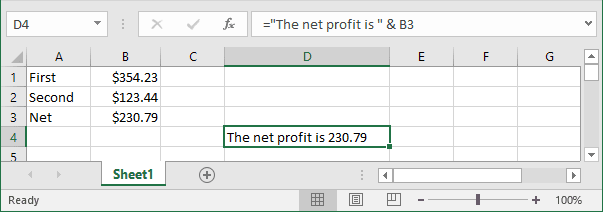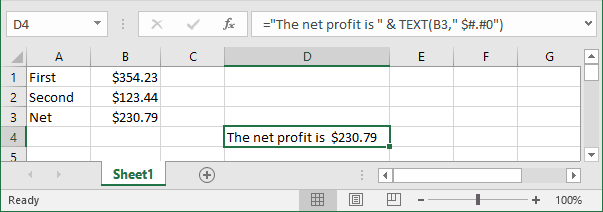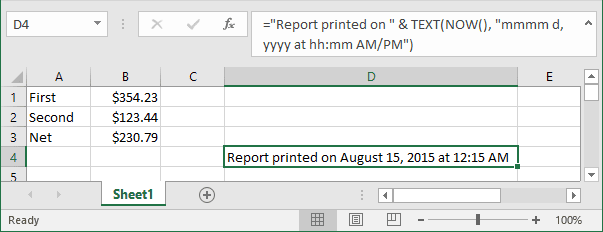# Combining text and formatted values

Excel
The Excel TEXT function enables you to specify numbers and date format.

Although this function may appear to have dubious value, it does serve some useful purposes.

For example, the formula in cell D4 is: = "The net profit is " & B3.This formula essentially combines a text string with the contents of cell B3 and displays the result. Note, however, that the contents of B3 are not formatted in any way. You might want to display the contents of B3 using a currency number format.

Note: Contrary to what you might expect, applying a number format to the cell that contains the formula has no effect. This is because the formula returns a string, not a value.

Here's a revised formula that uses the TEXT function to apply formatting to the value in B3: = "The net profit is " & TEXT (B3, " \$#.#0").

This formula displays the text along with a nicely formatted value:The second argument for the TEXT function consists of a standard Excel number format string. You can enter any valid number format string for this argument.

Here's another example that uses the NOW function (which returns the current date and time). The TEXT function displays the date and time, nicely formatted.

= "Report printed on "& TEXT (NOW (), " mmmm d, yyyy at hh:mm AM/PM").

The formula might display the following:See also this tip in French: Combiner du texte et valeurs formatées.